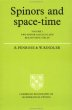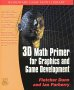# Maths - Books about Transformations TheoryRoger Penrose - The Road to Reality: Partly a 'popular science' book as it tries to minimise the number of equations (Not that I'm complaining much his book 'Spinors and space-time' went over my head in the first few pages) it still has lots of interesting results that its difficult to find elsewhere.Spinors and Space-Time: Volume 1, Two-Spinor Calculus and Relativistic Fields (Cambridge Monographs on Mathematical Physics) by Roger Penrose and Wolfgang Rindler - This book is about the mathematics of special relativity, it very quickly goes over my head by I hope I will understand it one day.Visual Complex Analysis - If you already know the basics of complex numbers but want to get an in depth understanding using an geometric and intuitive approach then this is a very good book. The book explains how to represent complex transformations such as the Möbius transformations. It also shows how complex functions can be differentiated and integrated.Geometric Algebra for Physicists - This is intended for physicists so it soon gets onto relativity, spacetime, electrodynamcs, quantum theory, etc. However the introduction to Geometric Algebra and classical mechanics is useful.Geometric Algebra for Computer Science: An Object-oriented Approach to Geometry. This book stresses the Geometry in Geometric Algebra, although it is still very mathematically orientated. Programmers using this book will need to have a lot of mathematical knowledge. Its good to have a Geometric Algebra book aimed at computer scientists rather than physicists. There is more information about this book here.

 metadata block see also: Correspondence about this page Book Shop - Further reading. Where I can, I have put links to Amazon for books that are relevant to the subject, click on the appropriate country flag to get more details of the book or to buy it from them.3D Math Primer - Aimed at complete beginners to vector and matrix algebra. Other Math Books

This site may have errors. Don't use for critical systems.